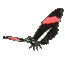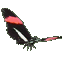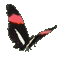Teaching Trigonometry Sine Functions - Fun Math Help - Game Tips:

The sine of angle A can be written as sin A and
sin A equals the ratio (y/r) = (4)/(5) when point D is (x,y) = (3,4) and r = AD = 5.

When angle A is in standard position at the origin, the sine ratio of A is (y coordinate)/(radius) = (4)/(5).
Using the Pythagorean Theorem, radius r=5 can be calculated from point D(3,4) as 32 + 42 = r2.

- When angle A is in standard position at the graph's origin (0,0) then sin A equals (y/r).
Since r is the hypotenuse of a right triangle, r can be found from point D(x,y) using x2 + y2 = r2 .
Consequently, sin A can also be described as (opposite side)/(hypotenuse).
sin(A) = o/h = y/r .

- Your Game Score is reduced by the number of butterfly hits.

- To slow the game speed repeat tap/click on the word Slider.
- To increase the game speed repeat tap/click on the word Math.
- Speed can also be adjusted with a keyboard's - and + keys.

- Refresh/Reload the web page to restart the game.